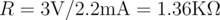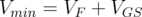Wiblocks --- FAQ

# Question

How do I use a JFET to regulate LED current?

With negative feedback a junction FET (JFET) can be used as a adjustable current regulator. The circuit on the right uses the resistor (R) to set the current through the LED. As long as the drain-to-source voltage (VDS) is above a minimum value a constant current flows through the LED.

### Calculation Example

The value of R is calculated using the "Drain Characteristics" curve available in the JFET datasheet. The graph shown is from the On-Semi BFR30LT1 datasheet.

Since the y-axis is drain current (ID) horizontal lines are constant current. For each gate-to-source voltage (VGS) there is a minimum drain-to-source voltage VDS above which ID is constant.

For example to maintain a current of 2.5mA a VGS of -3V is required. The resistance R would be --The minimum voltage for regulation isUsing an LED with a forward voltage (VF) of 2V and the BFR30LT1 at a VGS of -3V the minimum voltage for regulation is ≈ 5V. For voltages above 5V constant current is maintained.

A couple of other useful values can be read from the curves. VGS(OFF) is the value of gate-to-source voltage that forces the JFET drain current to zero (-5.8V for the BFR30LT1). The zero-gate-voltage drain current (IDSS) is the current that flows when VGS is zero (≈ 9mA for the BFR30LT1). IDSS is the maximum current of the JFET.